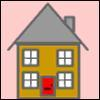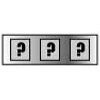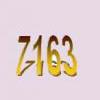Search by Topic

There are 5 NRICH Mathematical resources connected to Cube numbers, you may find related items under Numbers and the Number System.

Broad Topics > Numbers and the Number System > Cube numbersCubes Within Cubes

Age 7 to 14 Challenge Level:

We start with one yellow cube and build around it to make a 3x3x3 cube with red cubes. Then we build around that red cube with blue cubes and so on. How many cubes of each colour have we used?Smith and Jones

Age 14 to 16 Challenge Level:

Mr Smith and Mr Jones are two maths teachers. By asking questions, the answers to which may be right or wrong, Mr Jones is able to find the number of the house Mr Smith lives in... Or not!A Square Deal

Age 7 to 11 Challenge Level:

Complete the magic square using the numbers 1 to 25 once each. Each row, column and diagonal adds up to 65.Forgotten Number

Age 11 to 14 Challenge Level:

I have forgotten the number of the combination of the lock on my briefcase. I did have a method for remembering it...Some Cubes

Age 16 to 18 Challenge Level:

The sum of the cubes of two numbers is 7163. What are these numbers?